# Graphing with Functions Chapter Exam

Exam Instructions:

Choose your answers to the questions and click 'Next' to see the next set of questions. You can skip questions if you would like and come back to them later with the yellow "Go To First Skipped Question" button. When you have completed the practice exam, a green submit button will appear. Click it to see your results. Good luck!

### Page 1

#### Question 2 2. If the following graph is an implicit function, then how many real functions would it take to graph it?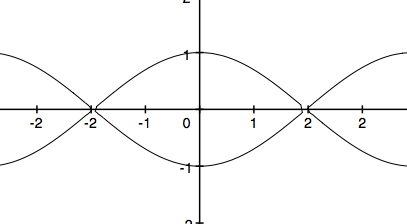### Page 3

#### Question 13 13. The implicit function x^2=y^2 is graphed below. Which functions give the same graph?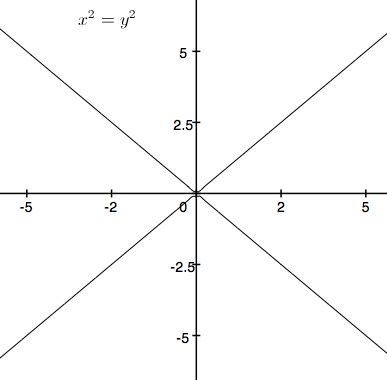### Page 5

#### Question 21 21. What is the slope of the line tangent to the curve given by the equation y = (x-1)²+2 at the point (1,2)?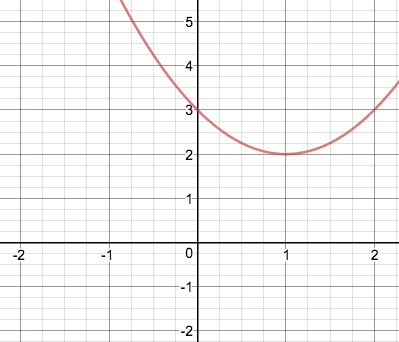#### Question 22 22. How many functions y=f(x) are needed to represent the graph below?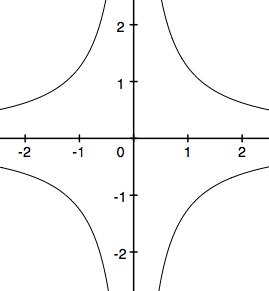### Page 6

#### Question 29 29. What are the asymptotes for this equation?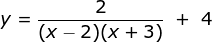#### Graphing with Functions Chapter Exam Instructions

Choose your answers to the questions and click 'Next' to see the next set of questions. You can skip questions if you would like and come back to them later with the yellow "Go To First Skipped Question" button. When you have completed the practice exam, a green submit button will appear. Click it to see your results. Good luck!

Support